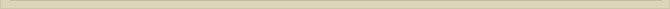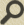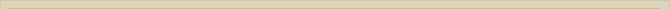## The photon and the constant of Planck (h)

When you are interested in physics you must read “Unbelievable“!

After the electron we discussed the photon. This was necessary to explain the observed time dilatation of cosmic particles. The stimulated electron and the possibility to emit the oscillation energy has been discussed. We however did not discuss the possible physical presentation of the photon. We have now more deducted information to be able to describe the photon. High energetic photons can split up and produce a positron and electron. The assumption that in a photon a separation of charge is established is not too far-fetched. How else can a positive and negative particle emerge from a photon? Photons with less energy probably also have a separation of charge during the oscillation, but the energy is insufficient to obtain two electrons. The charge of a positron is +Qe and of the electron –Qe. We assume that the charge separation in any arbitrary photon is +Qe/n and –Qe/n. The attracting force between the two separated charge +Qe/n and –Qe/n is: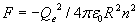with R the distance between both charge Qe/n.

The problem when energy is calculated from force x distance, is that one does not know until what distance the force is active. When R approaches zero the force between both charges becomes infinitely high and a singularity arises. When we discussed the proton and neutron we calculated the radius of both at Rp and Rn. We assumed that the fusion of positrons and electrons would stop when the radius of the compiled particle reached the radius of the point-volume. So we consider Rp and Rn to be an approximation of the radius of the point-volume. When both charges +Qe/n and –Qe/n approach and merge to one point-volume (R<=Rn), there is no external force in the ether to accumulate and concentrate energy anymore. The singularity is avoided.

We have the radius Rn as an indication to what distance the force is active. We however have no idea to what distance both charges separate in the oscillation. The wavelength of a photon λ decreases proportionally with the energy of the photon. To be able to integrate the force F between both particles we assume the arbitrary maximum distance of ¼ of the wavelength.

The still unknown factor is the value of n. We know the force and energy increases with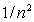, therefore the wavelength λ of the photon must be proportional with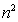. To solve the problem and to be able to calculate the energy of the oscillation we need the exact relation between λ and n. We know that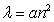, but we do not know the value of a. We however know that if n=1 the charge separation is +Qe and –Qe and that the radius of an electron is Re. The most direct and therefore most likely assumption for the relation betweenis a=Re. All factors to calculate the energy of the oscillation are known. We calculate for the energy of the oscillation :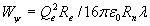With Re the radius of the electron, Rn the radius of the neutron, as an approximation for the radius of the point-volume, Qe the elementary charge of the electron and λ the wavelength of the photon. The calculated energy accounts only for the oscillation. The photon not only oscillates, but it travels also with the speed of light c. Assuming the energy of the photon is, like the energy in the electron, equally divided over both degrees of freedom, the total energy of the photon will be Wf=2Wψ (oscillation + kinetic energy).

The calculated energy of the oscillation, between two charges +Qe/n and –Qe/n, and the kinetic energy of the photon (c), is equal within 6% of the energy of a photon according to Wf=hv. The constant of Planck h is therefore theoretically accounted for within an error of approx. 6%. Again pure coincidence?

It is very unlikely that the constant of Planck is derived theoretically a simple and straightforward approach, if the calculated radius Rn is not related to h. The above derivation implicates that a photon can be described as an oscillation of two charges moving with speed c. Figure 21 illustrates the electromagnetic oscillation of both charges schematically.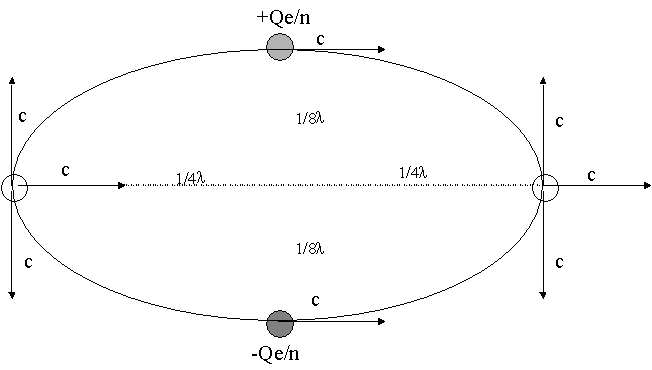Figure 21. The schematical illustration of the position of charges in the photon.

The arbitrary maximum distance between both charges was put on ¼λ. When the distance between both charges is ¼λ they reach maximum distance and do not separate any further. The oscillation energy is now fully electrostatic and equal to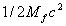; half the energy of the photon. When both charges approach each other within the point-volume the oscillation energy is transformed from potential to dynamic or in other words from electrostatic to magnetic/kinetic. The consequence of the presentation is that a photon can simply be described as an oscillation of two opposite charges. Each charge represents a mass of ½Mf; with Mf the mass equivalence of a photon. The oscillation is right-angled at the direction of the photon. The impulse of a photon, presented as two oscillating charges/masses, moving with speed c in the direction of movement, is If=Mfc. The impulse of the photon is therefore equal to the impulse I of any moving mass M with speed v (I=Mv). The impulse of the photon is equal to the impulse of any ordinary moving mass according to classical mechanics. This is so because half the energy/mass of the photon does not contribute to the impulse in the direction of movement. The oscillation does not show an impulse outside the oscillation.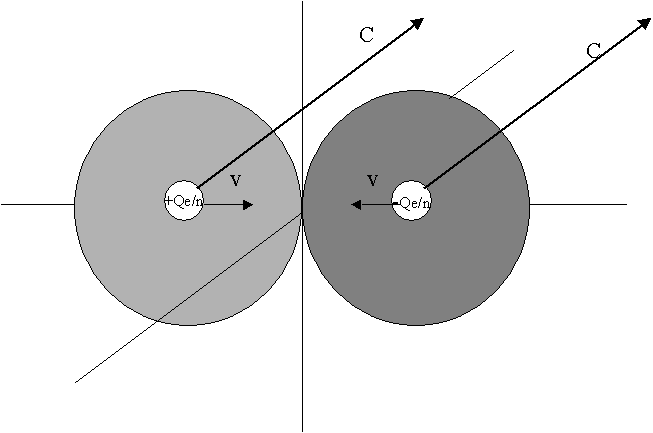Figure 22. The instant charge position and dielectric displacement of a photon.

When the charges approach each other the potential energy decreases and the magnetic energy increase. When both charges merge the oscillation energy is kinetic. The schematically complete cycle of the oscillation of a photon is presented in figure 23. The photon shows all its secrets in a simple straightforward classic mechanical description. This way there is nothing mysterious or relativistic about the photon. The ether makes the presentation simple and understandable and should not be rejected for that.

When the reader drives in a car with speed v, and the mass of the car is Ma, than the kinetic energy is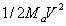. Imagine your car has two compartments, where both can move separately of each other. You are in one compartment and your passenger in the other.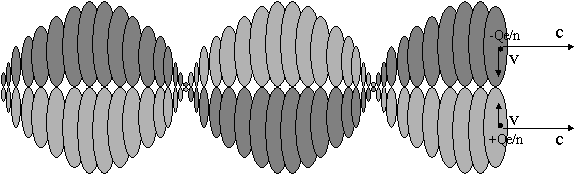Figure 23. The photon moving through the ether.

The car moves with speed v, and both compartments move with v opposite and right-angled with the speed of the car. You and your passenger pass each other with v in opposite direction right-angled at the direction of the car. Both compartments are held together with a spring, so the speed of the compartments diminishes when they separate. When you and your passenger stop separating the energy of the oscillation is potential. The spring pulls you together and you pass each other again with speed 2v. Now the energy of the oscillation is dynamic. The energy of the oscillation is also, so the total energy (speed + oscillation) of the moving and oscillating car is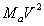and the impulse in the direction of movement is MaV: comparable with the photon. In the comparison only mass and speed differ.

Next chapter: The photon and the constant of Planck mathematically